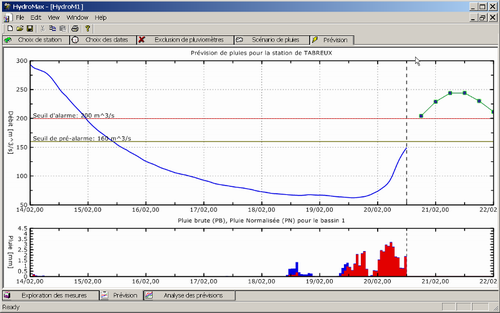## 1

`PGL` is a library that encapsulates plot capabilities in a MFC project for VC++ 6 and VC++ .NET 2003. It is designed to be able to easily plot data generated in a project without the need of any external software. In fact, with `CView` and ``` CDialog``` derived classes, you can have your app display chart in 5 minutes.

The aim of `PGL` is not to have a user-friendly environment but rather being able to generate any plot from the source code.

`PGL` was originally using `OpenGL` to raster graphics but now it uses `GDI+` (so you need to install Microsoft SDK to compile ``` PGL```).

## 2

1. Add the following in your StdAfx.h file :
```#include "PGL.h"
```
2. Since `PGL` is using `GDI+`, you must initialize it :
• Add the following variable to your `CWinApp` derived class
```ULONG_PTR m_ulGdiplusToken;
```
• Add the following to the CWinApp::OnInitInstance function to initialize `GDI+`
```// initialize <code>GDI+ (gdi+ is in Gdiplus namespace)
Gdiplus::GdiplusStartupInput gdiplusStartupInput;
Gdiplus::GdiplusStartup(&m_ulGdiplusToken, &gdiplusStartupInput,
NULL);```
• Add the following to the CWinApp::OnExitInstance function to clean up `GDI+`.
```// shutdown GDI+
Gdiplus::GdiplusShutdown(m_ulGdiplusToken);```

Your project should work fine now.

### Examples

All these examples are accessible in the source. See the example menu of TestPGL.

#### Example 1 : Drawing a simple line

This is a first explanatory example. We suppose that the points (x,y) of the line have been generated and are stored in two array `pX,pY` of size ``` nPoints``` ( note that you can also pass data as `std::vector<double>` to `PGL`).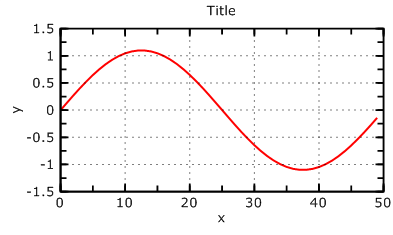Here's the code I used to generate the data: a simple sinusoid. Note that the y are in [-1.1, 1.1] but `PGL` will handle axe labelling the have nice units.

```// generate data
int nPoints = 50;
double* pX=new double[nPoints];
double* pY=new double[nPoints];
for (UINT i=0;i< nPoints;i++)
{
pX[i]=i;
pY[i]=sin(i/(double)nPoints*2*3.14)*1.1;
}```
1. First, create a graph object:
```CPGLGraph* pGraph = new CPGLGraph;
```
Note that you can check all `PGL` object with `ASSERT_VALID` since they all inherit from ``` CObject```.
2. Create a 2D line:
```CPGLLine2D* pLine = new CPGLLine2D;
```
3. Attach the data to the line. ``` PGL``` will handle the memory afterwards. That is, it will delete the pointers of data at the object destruction. This means `pX,pY` MUST have been allocated on the heap !
```pLine->SetDatas( nPoints /* number of points */,
pX /* x(i) */, pY /* y(i) */);
```
4. (Optional)Change some properties of the line: pLine->SetLineWidth(2);

5. Add the line to the graph (note that an object can be added to only one graph):
```pGraph->AddObject(pLine);
```
6. Make `PGL` scale the plot (automatically)
```pGraph->ZoomAll();
```
7. Create a dialog box and display the plot:
```CPGLGraphBitDlg graphdlg(this, pGraph);
graphdlg.DoModal();
```
You should have the same as the image above. Note that this image (PNG) has been generated by `PGL`.

Example 2 : Adding a line with level of detail control

You may have to plot line with thousands of points. This can become very heavy and especially if you export it to EPS, the files can become very large. To overcome this problem, you can use a line with LOD included in `PGL`.In this examples, we approximate the previous line. Starting from the previous example,
1. Change the line of code
```CPGLLine2D* pLine = new CPGLLine2D;
```
to
```CPGLLine2DLOD* pLine = new CPGLLine2DLOD;
```
2. Change tolerance of level of detail
```pLine->SetTol(0.05);
```
3. Shrink the number of points by a desired compression ratio (here to 10% with 2% threshold)
```pLine->ShrinkNorm(0.1,0.02);
```
On the figure above, you can see the original line and the approximated one. You can gain a serious amount of points using this technique!

#### Example 3: Customizing axis, labeling, etc...

As you can see in the previous image, all the parameters of the objects are changeable in the code. In this example, we shall
• change the title text,
• turn off horizontal grid,
• show right label,
• change number of ticks on the top axis,
• switch to time labelling for the x-axis,
• and more...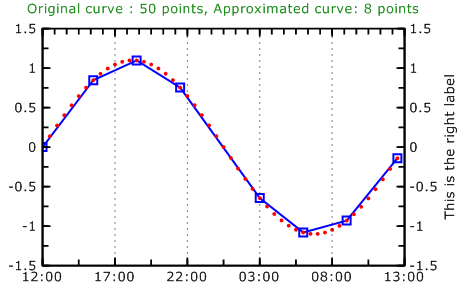We start from the second example and add the following line of code before calling ``` ZoomAll()```.
1. Get a pointer the axis object (there a huge mistake of English but in French it's ok :)(axe -> axis))
```CPGLAxe2D* pAxis = pGraph-&gtGetAxe();
```
2. Change the title text and color
```pAxis->SetTitle(str);
```
or
```pAxis->GetTitle()->SetString(str);
```
```pAxis->GetTitle()->SetColor(0 /* red */,0.5f /* green */,
0 /* blue*/ /* alpha optional */);
```
3. Turn off vertical grid, (vertical -> 0, horizontal -> 1)
```pAxis->SetShowGrid(1,FALSE);
```
4. Show and change right label,
```pAxis->GetRightLabel()-&gtShow(TRUE);
pAxis->GetRightLabel()-&gtSetString("This is the right label");
```
5. Show right numbering
```pAxis->GetRightNumber()->Show();
```
6. Changing number of ticks on the top axis,
```pAxis->SetTopSecondTicksNb(5);
```
7. Switch to time labelling the x-axis,
```// enable time labelling
pAxis->SetTimeLabel(TRUE);
// set origin, time step and format (see COleDateTime.Format for details)
pAxis->SetTimeLabelFormat(COleDateTime::GetCurrentTime()
/* Time at zero. */,
COleDateTimeSpan(0,0,30,0) /* Time per unit */,
"%H:%M:%S" /* String format */);
```
I've also disabled the line drawing and set the tolerance to 0.025 for the LOD line. Of course, you can do much more. This is just an example.

#### Example 4: Sub-plotting !

What about putting multiple plots on a figure: that's possible in `PGL` in many ways. In fact you can add plots to plots, and so on.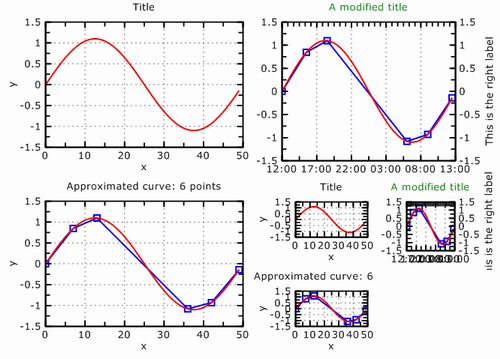The class `CPGLGraph` is inherited from a generic plot class : ``` CPGLRegion```. You can either
• use the function `Divide(m,n)` to divide the region in an array of m rows and n columns (Note that this method erase all object in the region). After that, you can access the elements with `GetChilds(i)` (the regions are created row by row). You can get the number of children with ``` GetNChilds()```:
```// allocated somewhere
CPGLRegion* pRegion;
// dividing
pRegion->Divide(m,n);
// accessing region at row 2 and column 1 (zero based index)
CPGLRegion* pChildRegion = pRegion->GetChild(2*n+1);
```
• Create an add directly a region using `AddRegion`. To use this method you must ``` SetNormBBox(...)``` to set the bounding box (in Normalized coordinates with respect to the parent region)
```CPGLRegion* pChildRegion = pRegion->AddRegion();
pChildRegion->SetNormBBox(0.1 /* llx */ , 0.2 /* lly */ ,
0.7 /* urx */ , 0.8 /* ury */);
```
Of course, you can divide child regions and so on.

#### Example 5: Changing properties of objects at run time

You can explore the object hierarchy by right clicking the view or dialog. Unfortunately, serialization is not working yet. So it is lost work...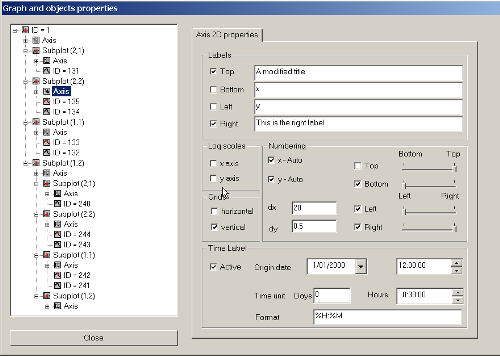## 3

The documentation is generated with Doxygen and Doxygen studio. See Plot Graphic Library.dow file. Otherwize, it is shipped with the Microsoft Installer.High-speed Charting Control, Real Time Chart, Data Visualization, C# Source Code